September 1, 2023

# Linear Algebra Courses

These Linear Algebra Courses relate to the subjects of data science and machine learning effectively.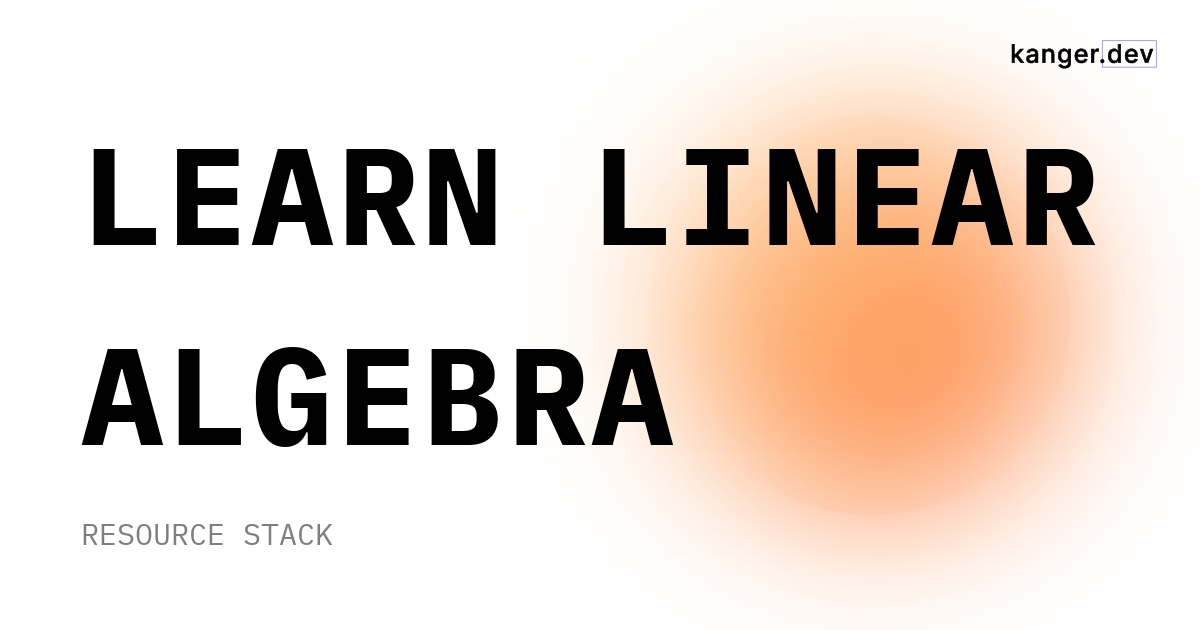Essential
Introductory

Linear Algebra is a vital cog in data scientists' skill set, but learners often neglect it because they find maths intimidating.

Whether you're looking to launch a career in AI or simply dip your toes into the world of Machine Learning, this stack will help you determine which Linear Algebra course best aligns with your experience level.

## 3Blue1Brown: Essence of Linear Algebra

High-valueThis is probably one of the best courses on the internet. It lays out the foundations of linear algebra using a distinctive animation-and-visuals style.

Beginner
Course
Beginner
Course

## Algebra: Elementary to Advanced Specialization

RecommendedThe best thing about this specialization is its comprehensive and beginner-friendly curriculum, specifically designed to equip individuals with essential data science and machine learning skills.

Beginner
Specialization
Beginner
Specialization

## Linear Algebra for Machine Learning and Data Science

High-ROI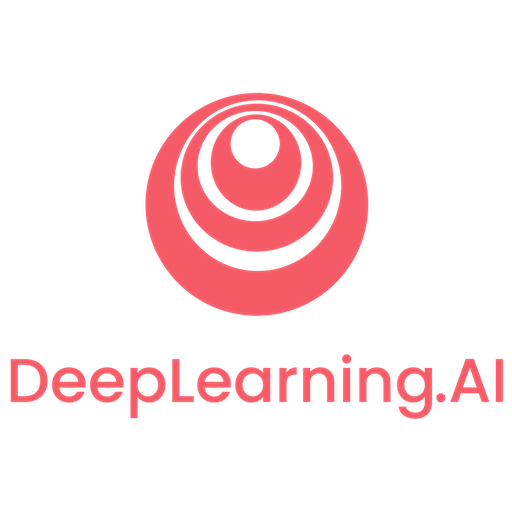This intermediate-level course will enhance your understanding of vector and matrix algebra, linear transformations, and eigenvalues, enabling you to apply these concepts to machine learning problems effectively.

Beginner
Specialization
Beginner
Specialization

## Linear Algebra for Data Science in R

Good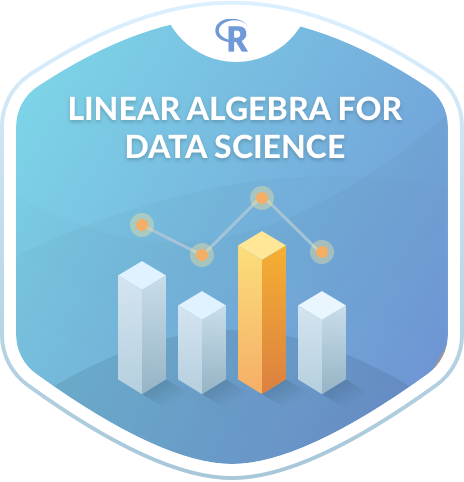This linear algebra course integrates math and R programming, covering vectors, matrices, matrix-vector equations, eigenvalue/eigenvector analyses, and dimension reduction using principal component analysis on real-world datasets, making it ideal for data science beginners with basic math knowledge.

Beginner
Course
Beginner
Course

## Mathematics for Machine Learning: Linear Algebra

Long-running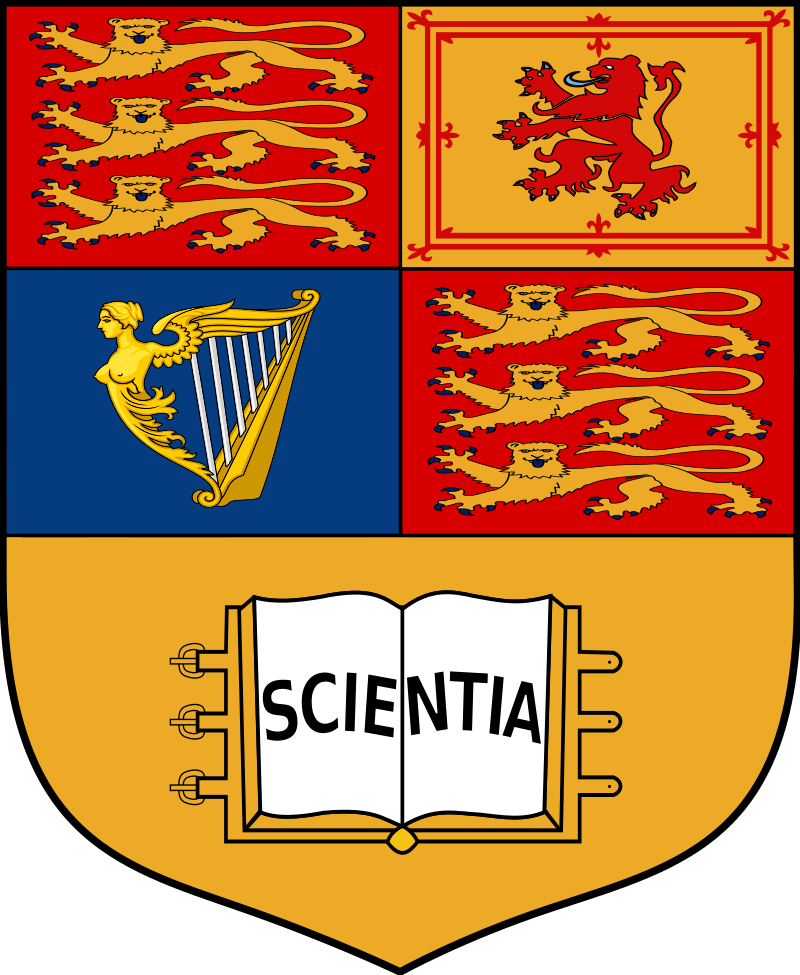This course is jam-packed with lessons and exercises to help you develop an intuitive understanding of linear algebra concepts such as vectors, matrices, basis (linear algebra), eigenvalues, eigenvectors, and transformation matrices, among others.

Beginner
Course
Beginner
Course

## Linear Algebra for Data Science Using Python Specialization

GoodThis course provides an excellent introduction to Python for beginners, building upon their previous mathematical foundations in data science.

Beginner
Specialization
Beginner
Specialization

## Applying Linear Algebra with R

Good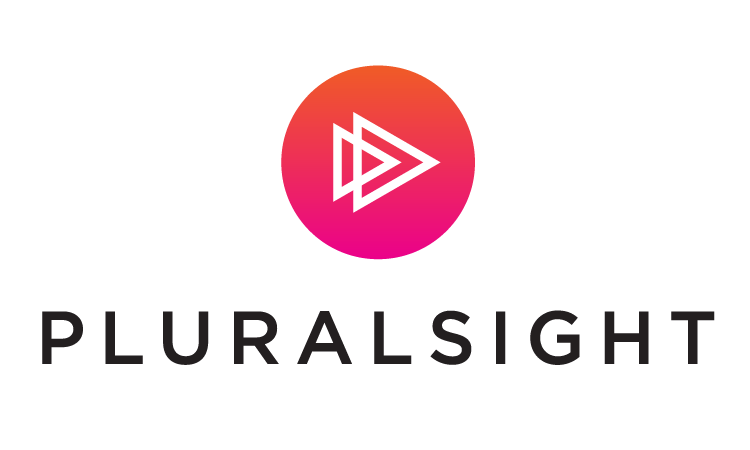This intense course focuses on learning the math behind predictive models, covering topics such as basic matrix arithmetic, advanced matrix mathematics, linear regression, and other machine learning concepts.

Intermediate
Course
Intermediate
Course

## Become a Linear Algebra Master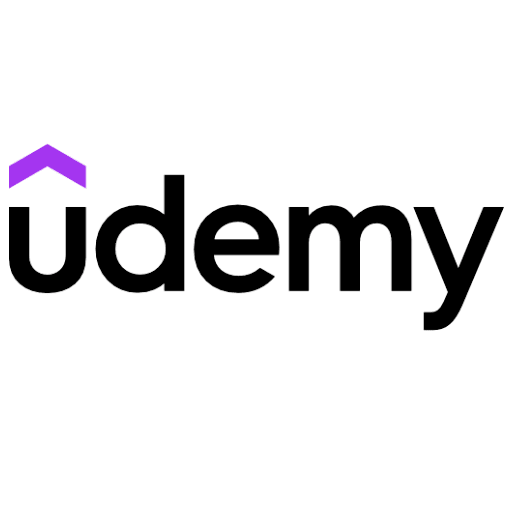This beginner-friendly course promises to teach you the fundamentals of Linear Algebra and then test your knowledge with over 400 practice questions.

Beginner
Course
Beginner
Course

## Linear Algebra For Machine Learning

Underrated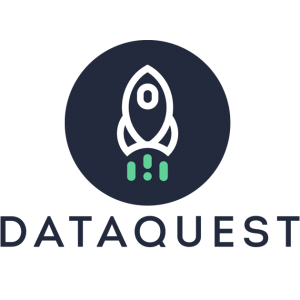You will learn the linear algebra concepts, such as neural networks and backpropagation, that underlie machine learning systems and enable the training of deep learning neural networks.

Intermediate
Course
Intermediate
Course

## Complete linear algebra: theory and implementation in code

UpdatedThis course teaches concepts in linear algebra and matrix analysis, and demonstrates their implementation in MATLAB and Python.

Beginner
Course
Beginner
Course
No items found.
No items found.

Linear Algebra is one of the foundational blocks of Data Science and Machine Learning. It's hard to think of algorithms or applications that aren't implemented using linear algebra.

Programming languages like Python, R, and Julia offer a plethora of packages for working with data to solve complex problems by applying linear algebra. You'll be using linear algebra to perform the required calculations and analyses efficiently. It is used for Principal Component Analysis to reduce the dimensionality of data. It is also practical for working with neural networks, as well as the representation and processing of the network.

With a functional knowledge of Linear Algebra, you will develop a better intuition for ML and deep learning algorithms. It is your key to unlocking a whole new world — you will also be able to code algorithms from scratch and make your own variations to them.

Linear Algebra can be difficult for beginners, but you can punctuate your efforts to learn the prerequisites, internalize the basics, and then build from there. For effective learning, get a good book and check out adjunctive resources to become highly prepared for learning advanced topics.AI Disclaimer: The following text is generated by an AI model developed by OpenAI. While efforts have been made to ensure the accuracy and coherence of the content, there is a possibility that the model may produce hallucinated or incorrect information. Therefore, we strongly recommend independently verifying any information provided in this text before making any decisions or taking any actions based on it.

Still wondering whether learning Linear Algebra is of any value? Read on for answers to frequently asked questions about Linear Algebra for Data Science and Machine Learning courses.

Is Linear Algebra fundamental for Data Science?

The short answer is yes, it is foundational for data science. Linear algebra is the language of algorithms that powers diverse data science algorithms and popular applications like natural language processing and computer vision. If you're not aiming for low-hanging fruit, consider linear algebra a must-know subject in data science for a rewarding career.

Is Linear Algebra Hard to Learn?

The basics are not very difficult, but it can be challenging for absolute beginners. The obstacle for many learners is finding the right course that teaches terms and definitions, as well as how to determine the type of calculation and analysis needed to obtain the required results. It also depends on whether you are learning Linear Algebra for data science or machine learning.

Is Linear Algebra Necessary for Data Science?

To become a competent Machine Learning Engineer/Scientist or a Data Scientist, you need to know linear algebra and optimization theory. Understanding linear algebra is a fundamental aspect of machine learning (ML). Areas of mathematics essential for machine learning, such as calculus, require prior knowledge of linear algebra, which will help you understand ML to a greaterextent.

What is the best Linear Algebra Course for Data Science?

These Linear Algebra courses are designed to take you from a total novice to a skilled ML practitioner, focusing on both Linear Algebra and its practical applications. It is important to know which math you need to learn and become equipped with a working knowledge for studying advanced Machine Learning topics. You will learn the fundamentals with programming (Python/R) to become a trained practitioner, but you should not expect to become a scientist overnight. It takes burning some midnight oil.

What Else Can You do to Learn Algebra for Data Science?

In addition to a Linear Algebra course, you can improve your algebra skills by watching YouTube tutorials, practicing with books, exploring different tools, and reading blogs. Check out our Math Stack to discover resources and introductory study guides that explain how to learn in-demand math skills.

Reference/ Credits
• FlatIcon
No spam. In-depth analysis, expert opinions, startup perks, and resources to bootstrap your growth.